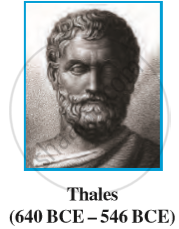# Concept for Euclid’S Geometry

## Notes

The word ‘geometry’ comes form the Greek words ‘geo’, meaning the ‘earth’, and ‘metrein’, meaning ‘to measure’.
A Greek mathematician, Thales is credited with giving the first known proof. This proof was of the statement that a circle is bisected (i.e., cut into two equal parts) by its diameter.One of Thales’ most famous pupils was Pythagoras.  Pythagoras and his group discovered many geometric properties and developed the theory of geometry to a great extent.This process continued till 300 BCE. At that time Euclid, a teacher of mathematics at Alexandria in Egypt, collected all the known work and arranged it in his famous treatise called ‘Elements’. He divided the ‘Elements’ into thirteen chapters, each called a book. These books influenced the whole world’s understanding of geometry for generations to come.

If you would like to contribute notes or other learning material, please submit them using the button below.

### Shaalaa.com

Introduction of Euclid Geometry [00:17:04]
S
0%